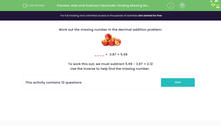# Add and Subtract Decimals: Finding Missing Numbers

In this worksheet, students practise their decimal addition facts by using subtraction to find the missing number.Key stage:  KS 2

Curriculum topic:   Number: Fractions, Decimals and Percentages

Curriculum subtopic:   Solve Problems with Decimal Places

Popular topics:   Decimals worksheets

Difficulty level:#### Worksheet Overview

Work out the missing number in the decimal addition problem:____ +  3.87 = 5.99

To work this out, we must subtract 5.99 - 3.87 = 2.12

Use the inverse to help find the missing number.

### What is EdPlace?

We're your National Curriculum aligned online education content provider helping each child succeed in English, maths and science from year 1 to GCSE. With an EdPlace account you’ll be able to track and measure progress, helping each child achieve their best. We build confidence and attainment by personalising each child’s learning at a level that suits them.

Get started